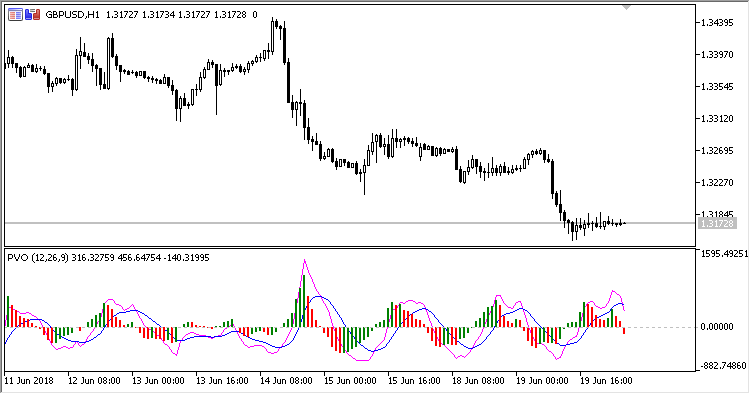# PVO – indicator for MetaTrader 5

• A+
category：MT5 INDICATORS

Indicator PVO (Percentage Volume Oscillator) represents a momentum for the volume. It calculates the difference in the percents of large MA between two moving averages based on volumes.

It has ten configurable parameters:

• Show histogram - whether to show the histogram (Yes/No);
• Show PVO - whether to show the PVO line (Yes/No);
• Show Signal - whether to show the signal line (Yes/No);
• Fast MA period - fast MA calculation period;
• Fast MA method - fast MA calculation method;
• Slow MA period - slow MA calculation period;
• Slow MA method - slow MA calculation method;
• Signal period - signal line calculation period;
• Signal method - signal line calculation method;
• Calculation mode - calculation mode:
• Absolute - in absolute values;
• Relative - in relative values.

Calculations:

```Signal = MA(PVO, Signal period, Signal method)
Histogram = PVO - Signal
```

Calculation mode Absolute:

`PVO = ShortMA - LongMA`

Calculation mode Relative:

`PVO = (FastMA - SlowMA) / SlowMA`

where:

```FastMA = MA(Volume, Fast MA period, Fast MA method)
SlowMA = MA(Volume, Slow MA period, Slow MA method)
```

Volume - tick volume